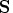Previous Page | Next Page

 Language Reference

## NCOL Function

finds the number of columns of a matrix

NCOL( matrix)

where matrix is a numeric or character matrix.

The NCOL function returns a single numeric value that is the number of columns in matrix. If the matrix has not been given a value, the NCOL function returns a value of 0.

For example, to let B contain the number of columns of matrix, use the following statement:

```
b=ncol(s);
```Previous Page | Next Page | Top of Page